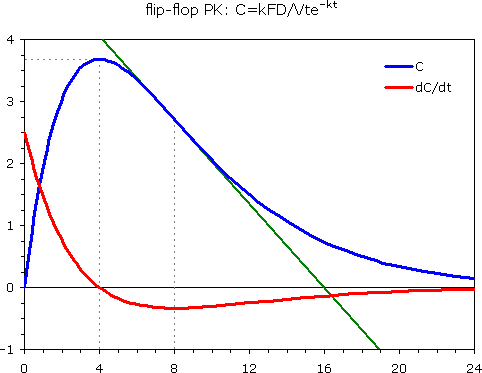jag009
★★★

NJ,
2013-09-13 15:48

Posting: # 11492
Views: 3,327

## Maybe a simple question? [PK / PD]

Hi all,

Can someone explain to me the following (or a kick in the butt if this is too simple?) from Csizmadia & Endrenyi? I don't have the Gibaldi PK book on hand (they referenced it from his book).

Basis of the Proposed Methods. If a drug exhibits first-order absorption with one-exponential disposition and the absorption (ka) and elimination (k) rate constants are equal, then the plasma concentration (C) depends on time according to:

C=kAtexp(-kt), where A=FD/V

For the above model, k=1/Tmax, where Tmax is the time when the plasma concentration reaches maximum.

How did he arrive to k=1/Tmax?

Thanks
John
___________________________________________________________________________
Csizmadia F, Endrenyi L. Model-Independent Estimation of Lag Times with First-Order Absorption and Disposition. J Pharm Sci Vol. 87:5. May 1998
Helmut
★★★Vienna, Austria,
2013-09-13 16:16

@ jag009
Posting: # 11494
Views: 3,178

## flip-flop PK

Hi John,

after administration of an IR formulation (if we have no IV data in the same subject) we assume that ka > kel (absorption is faster than elimination). In a controlled release formulation we try to slow down the absorption process. We reach flip-flop PK if ka = kel. Note that in the common formula we have FDka in the numerator and V(ka–kel) in the denominator. FDka divided by zero? Oops! Therefore, we use the given modification. Note, that in Phoenix/WinNonlin for the same reason we have Model #5 (and #6 with a lag-time).

» How did he arrive to k=1/Tmax?

Calculus followed by curve sketching and a little algebra. Start with the first derivative of the con­cen­tra­tion-time curve. Its root is at tmax (concentrations increasing before tmax = positive slope, decreasing after tmax = negative slope). Step-by-step:
The flip-flop models is

(1) C = kFD/Vtℯ–kt

The first derivative is

(2) dC/dt = kFD/Vℯ–kt – k2tFD/Vℯ–kt

We know that at tmax dC/dt = 0. Therefore, we can write

(3) kFD/Vℯ–ktmax = k2tmaxFD/Vℯ–ktmax

Solving for tmax (shortening some stuff and dividing by k), we get

(4) k = k2tmax
(5) 1 = ktmax and finally
(6) tmax = 1/k

Rearranging (6) gives the k = 1/tmax you quoted above.

Example: k 0.25, F 1, D 100, V 10k = 1/4 = 0.25, Q.E.D.
BTW, like in the usual one-compartment model the inflection point – where the tangent chances the “side” – is at the minimum of slopes (or the root of the 2nd derivative) = 2×tmax.
If you go for a semilog-plot you will notice that we have no linear phase. Therefore, in PK modeling we have a problem. If we aim for a one-compartment model and get similar estimates of ka and kel (generally with large variances and overlapping CIs) it’s time to try a flip-flop model.

» I don't have the Gibaldi PK book on hand.

An overrated book, IMHO. Gibaldi/Perrier work most of the time with decadic logarithms and therefore have to introduce this stupid t½ = 2.303/k… Not quite elegant didactics.

Cheers,
Helmut SchützThe quality of responses received is directly proportional to the quality of the question asked. 🚮
Science Quotes
jag009
★★★

NJ,
2013-09-13 19:51

@ Helmut
Posting: # 11495
Views: 2,666

## flip-flop PK

Thanks HelmutIng. Helmut Schütz